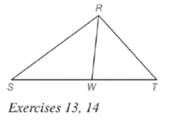Chapter 5.6, Problem 14EElementary Geometry For College St...

7th Edition
Alexander + 2 others
ISBN: 9781337614085

Solutions

Chapter
SectionElementary Geometry For College St...

7th Edition
Alexander + 2 others
ISBN: 9781337614085
Textbook Problem

Given: R W → bisects ∠ S R T Do the following equalities hold? a) R S S W = R T W T b) m ∠ S = m ∠ TTo determine

a)

To check: Whether the equality RSSW=RTWT holds or not.

Explanation

Given:

RW bisects SRT.

Theorem used:

Angle bisector theorem:

If a ray bisects one angle of a triangle, then it divides the opposite side into segments whose lengths are proportional to the lengths of the two sides that form the bisected angle.

Calculation:

Given: RW bisects SRT

To determine

b)

To check: Whether the equality mS=mT holds or not.

Still sussing out bartleby?

Check out a sample textbook solution.

See a sample solution

The Solution to Your Study Problems

Bartleby provides explanations to thousands of textbook problems written by our experts, many with advanced degrees!

Get Started

Use an inverse matrix to solve

Mathematical Applications for the Management, Life, and Social Sciences

In Exercises 13 and 14, find the inverse function of f. f(x)=4x+6

Calculus: An Applied Approach (MindTap Course List)

Evaluate the indefinite integral. dxax+b(a0)

Single Variable Calculus: Early Transcendentals

ex(ex)2 = a) ex3 b) e3x c) 3ex d) e4x

Study Guide for Stewart's Single Variable Calculus: Early Transcendentals, 8th